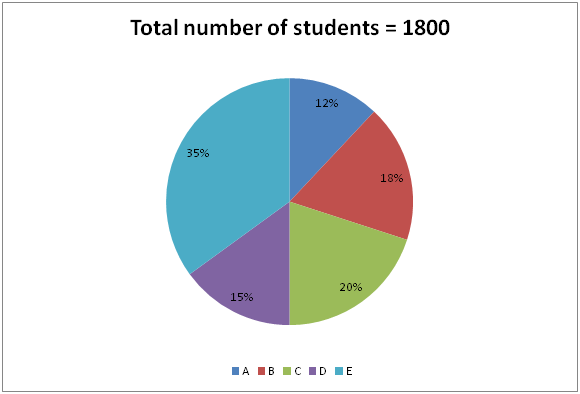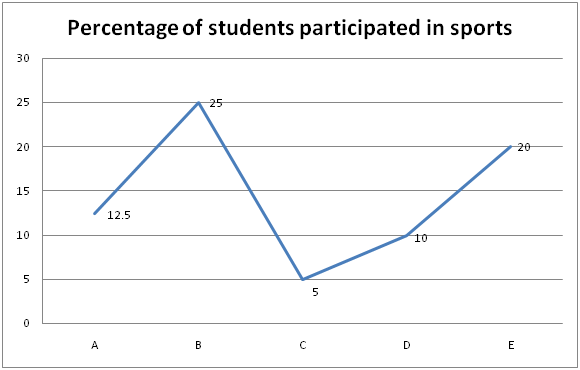# SBI Clerk Mains Quantitative Aptitude (Day-16)

Dear Aspirants, Our IBPS Guide team is providing new series of Quantitative Aptitude Questions for SBI Clerk Mains 2020 so the aspirants can practice it on a daily basis. These questions are framed by our skilled experts after understanding your needs thoroughly. Aspirants can practice these new series questions daily to familiarize with the exact exam pattern and make your preparation effective.

Start Quiz

Ensure Your Ability Before the Exam – Take SBI Clerk 2020 Mains Free Mock Test

Data Interpretation

Directions (1 – 5): Study the following information carefully and answer the questions given below?

Pie chart shows the percentage of students in each class and line graph shows the percentage of students participated in sports.1) What is the ratio of the number of students from E to the students participated in sports from C & D?

a) 14: 1

b) 1: 14

c) 13: 21

d) 21: 13

e) None of these

2) What % is the total number of students participated in sports from all the classes together?

a) 15.5%

b) 17.8%

c) 20.3%

d) 13.5%

e) None of these

3) Students participating in sports from C & D together is how much % more/less than the no. of students in A?

a) 71.72%

b) 73.15%

c) 79.16%

d) 76.18%

e) None of these

4) What is the difference between the no. of students participating sports in A & B together and the total no. of students in D & E together?

a) 792

b) 996

c) 874

d) 926

e) None of these

5) In which of the following, the no. of students participating sports is higher?

a) A

b) E

c) B

d) C

e) None of these

Inequality

Directions (6 – 10): Each question contains a statement followed by Quantity I, quantity II and Quantity III.

6) Quantity I: ∛8000000

Quantity II: In a shop, the ratio of the number of Hamam soap and Pears soap is 8:5. If there are 650 soaps in total, what is the difference between them?

Quantity III: A and B invest in the ratio of 2: 3. If 5% of the total profit is given to a charity and A’s share is 76, what is the total profit

a) Quantity I > Quantity II > Quantity III

b) Quantity I = Quantity II > Quantity III

c) Quantity I = Quantity III > Quantity II

d) Quantity I = Quantity II = Quantity III

e) None of these

7) Quantity I: x% of150 + 25% of 80 = 12.5% of 400. What is x?

Quantity II: Ratio of ages of A and B is 2: 3 and that of B and C is 2: 3. The sum of their ages is 57. How old is A?

Quantity III: If the numerator increased by 20% and the denominator is increased by 25%, the value becomes 240/275. What is the value of numerator?

a) Quantity I > Quantity II > Quantity III

b) Quantity I = Quantity II > Quantity III

c) Quantity I = Quantity II > Quantity III

d) Quantity I = Quantity II = Quantity III

e) None of these

8) Quantity I: The length and breadth of a rectangle is in the ratio of 3: 2. If the area is 216, what is the length?

Quantity II: √(5 + √49 + √16) =?

Quantity III: If 80% of a number is added to 80, then the result is the number itself. What is the 2% of the number?

a) Quantity I > Quantity II > Quantity III

b) Quantity I = Quantity II > Quantity III

c) Quantity I > Quantity II < Quantity III

d) Quantity I = Quantity II = Quantity III

e) None of these

9) Quantity I: 10, _, 27,85,345,1731

Quantity II: The black and red pen in the box is in the ratio of 2: 3. If 5 black pens are added and 4 red pens are removed from the box, the ratio becomes 17: 14. No. of black pens initially was _.

Quantity III: A sum ___ is invested in SI and it amounts to Rs.26 in 3 years and to Rs.28 in 4 years

a) Quantity I = Quantity II < Quantity III

b) Quantity I = Quantity II > Quantity III

c) Quantity I = Quantity III > Quantity II

d) Quantity I = Quantity II = Quantity III

e) None of these

10) Quantity I: x2 – 24x + 144 = 0

Quantity II: 405 sweets are distributed among children. No. of sweets received by each child is 20% of the total children. How many sweets did each child receive?

Quantity III: If 12 men can do a work in 5 days then to complete the work in 3 days how many men will be required?

a) Quantity I > Quantity II > Quantity III

b) Quantity I > Quantity II < Quantity III

c) Quantity I = Quantity III > Quantity II

d) Quantity I = Quantity II = Quantity III

e) None of these

Directions (1 – 5):Required ratio = 630: 45 => 126: 9 => 14: 1

Required percentage = 279/1800 * 100 = 15.5%

Required percentage = [(18 + 27) – 216]/216 * 100

= 171/216 * 100

= 79.16%

Required difference = 900 – 108 = 792

A – 27

B – 81

C – 18

D – 27

E – 126

Class E has maximum number of students participating in sports.

Directions (6-10) :

Quantity I: 200

Quantity II: 3 * 650/13 = 150

Quantity III: 5 * 76/2 = 190 = > profit = 190/95 * 100 = 200

Hence, Quantity I = Quantity III > Quantity II

Quantity I: x% of 150 + 20 = 50 = > x = 20

Quantity II: A: B: C = 4: 6: 9

19 – > 57 so 4 – > 12

A’s age = 12 years

Quantity III: [N * (120/100)]/[D * (125/100)] = 240/275

N/D = 10/11, N = 10

Hence, Quantity I > Quantity II > Quantity III

Quantity I: 2x * 3x = 216

x2 = 36 = > x = 6

3x = 18

Quantity II: √(5 + √49 + √16) =?

√(5 + 7 + 4) = ?

√16 =?

4 =?

Quantity III:

80/100 * x + 80 = x

4/5 x + 80 = x

x = 400

2% of x = 8

Hence, Quantity I > Quantity II < Quantity III

Quantity I: 10 * 1 + 2 = 12

12 * 2 + 3 = 27

27 * 3 + 4 = 85

85 * 4 + 5 = 345

345 * 5 + 6 = 1731

Quantity II: (2x + 5)/(3x – 4) = 17/14

28x + 70 = 51x – 68

23x = 138

x = 6

No. of black pens initially in the box = 2 * 6 = 12

Quantity III: 1 year = Rs.2, 3 years = Rs.6

Required sum = 26 – 6 = Rs.20

Hence, Quantity I = Quantity II < Quantity III

Quantity I: 12, -12 so 12

Quantity II: x * (20% of x) = 405, x = 45

20% of x = 9

Quantity III: 12 * 5 = 3 * x = > x = 20

Hence, Quantity I > Quantity II < Quantity III

 Check Here to View SBI Clerk Mains 2020 Quantitative Aptitude Questions Day 15 Day 14 Day 13 Click Here for SBI Clerk 2020 – Detailed Exam Notification
5 1 vote
Rating